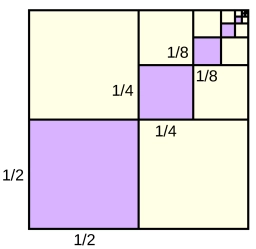# Simple coefficient

Find the value of k; if x = 3, y = 2 is a solution of the equation 2x + 3y = k.

k =  12

### Step-by-step explanation:Did you find an error or inaccuracy? Feel free to write us. Thank you!

Tips for related online calculators
Do you have a linear equation or system of equations and looking for its solution? Or do you have a quadratic equation?